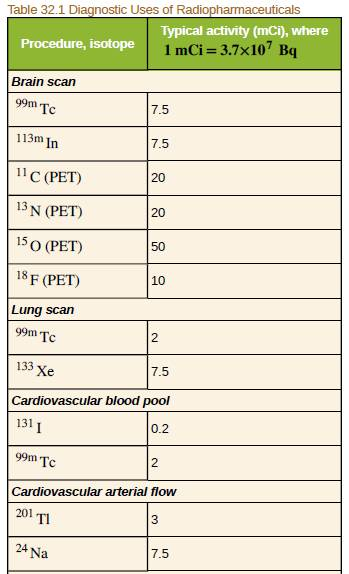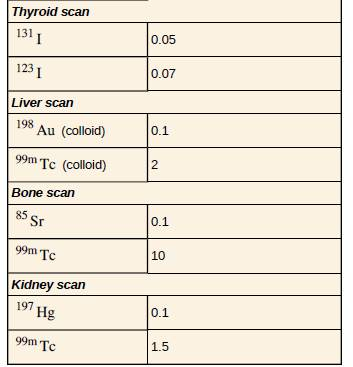Chapter 32, Problem 7PE

Chapter
Section
Textbook Problem

(a) Neutron activation of sodium, which is 100% 23Na, produces 24Na, which is used in some heart scans, as seen in Table 32.1. The equation for the reaction is 23 Na + n → 24 Na + γ . Find its energy output, given the mass of 24Na is 23.990962 u.(b) What mass at 24Na produces the needed 5.0−mCi activity, given its half−life is 15.0 h?To determine

(a)

The energy output of given reaction.

Explanation

Given:

The masses of N23a , N24a and n are

N23a=22.989769uN24a=23.990961un=1.008665u.

Formula used:

The formula used to calculate energy output is

E=Δm·c2

To determine

(b)

The mass of the technetium.

Still sussing out bartleby?

Check out a sample textbook solution.

See a sample solution

The Solution to Your Study Problems

Bartleby provides explanations to thousands of textbook problems written by our experts, many with advanced degrees!

Get Started